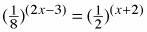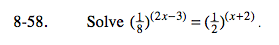Home > A2C > Chapter 8 > Lesson 8.1.4 > Problem8-58

8-58.

Solvefor x. Homework Help ✎Find a common base, then rewrite each side.
Set the exponents equal to each other and solve.

The common base is 2:

$\frac{1}{8} = 2^{-3} \text{ and } \frac{1}{2} = 2^{-1}$